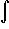#Interactive Real Analysis

Next | Previous | Glossary | Map

## 7.4. Lebesgue Integral

### Definition 7.4.17: Lebesgue Integral of Non-Negative Functions

If f is a non-negative measurable function defined on E and h is a bounded measurable function such that m( {x: h(x) # 0} ) is finite, then we defineE f(x) dx = sup{E h(x) dx, hf }
IfE f(x) dx is finite, then f is called Lebesgue integrable over E.
Next | Previous | Glossary | Map